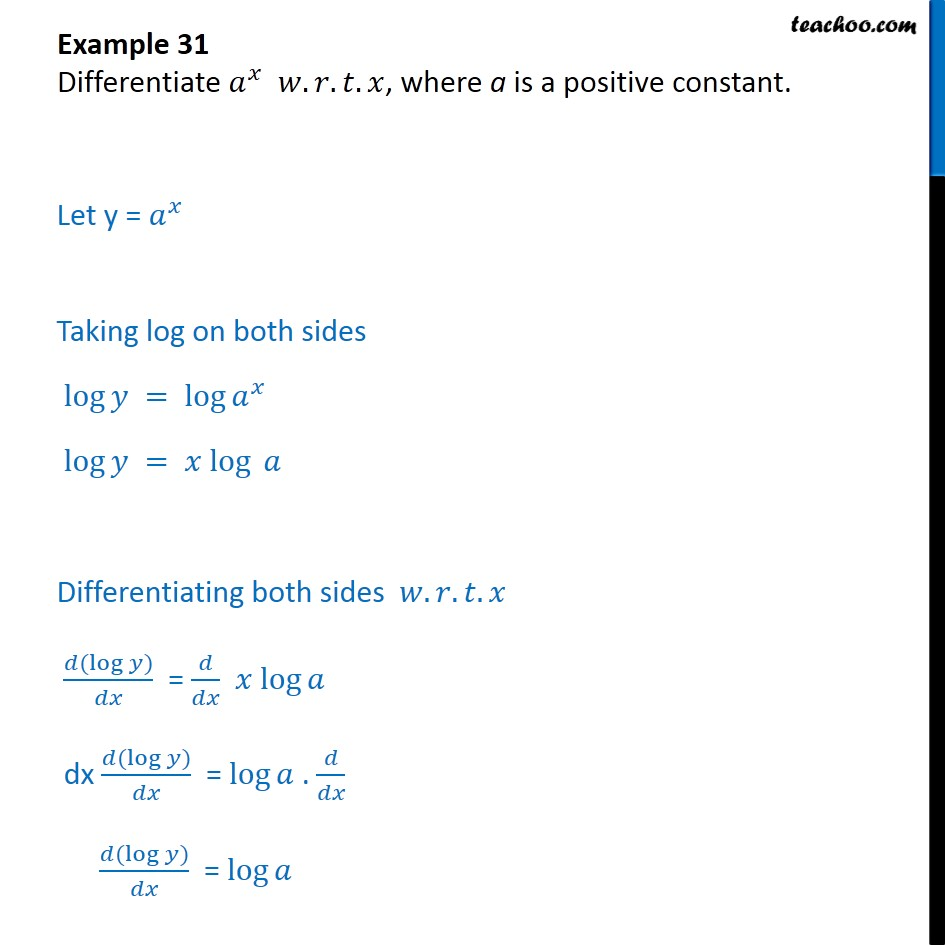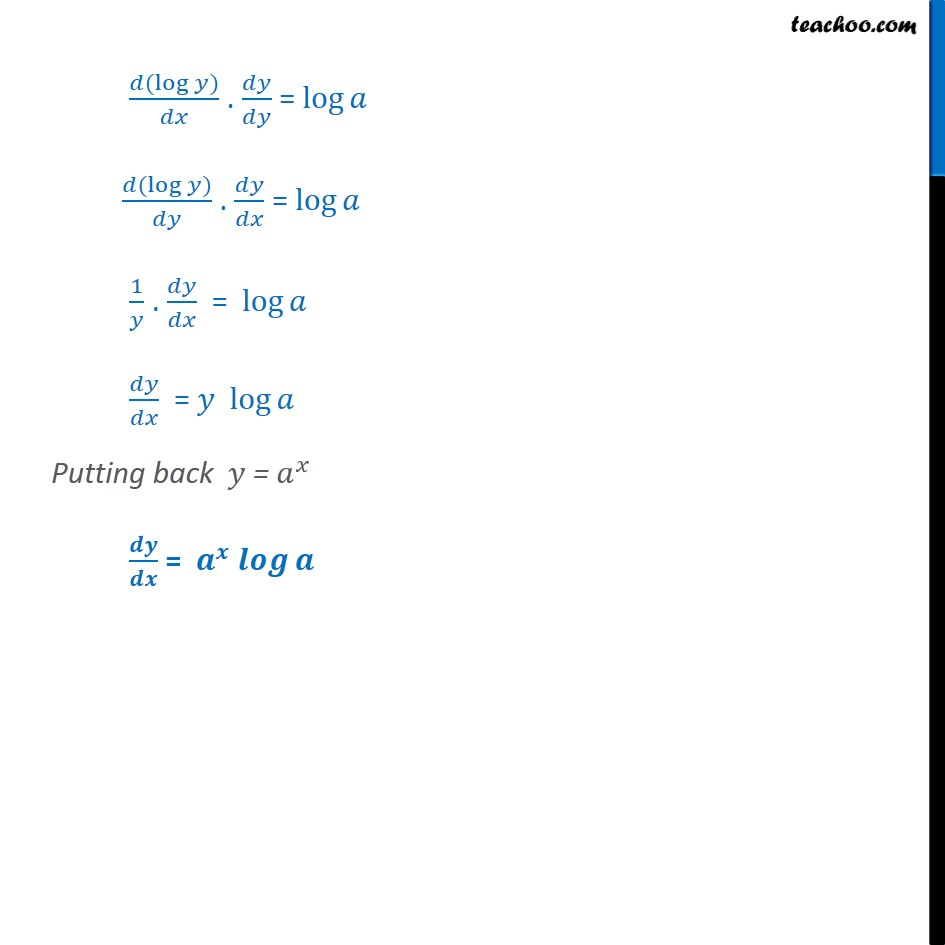1. Chapter 5 Class 12 Continuity and Differentiability
2. Serial order wise
3. Examples

Transcript

Example 31 Differentiate ^ . . . , where a is a positive constant. Let y = ^ Taking log on both sides log = log ^ log = log Differentiating both sides . . . ( (log ))/ = / log dx ( (log ))/ = log . / ( (log ))/ = log ( (log ))/ . / = log ( (log ))/ . / = log 1/ . / = log / = log Putting back = ^ / = ^

Examples